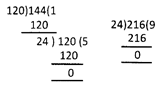Courses

# Test: Playing With Numbers - 3

## 20 Questions MCQ Test Mathematics (Maths) Class 6 | Test: Playing With Numbers - 3

Description
This mock test of Test: Playing With Numbers - 3 for Class 6 helps you for every Class 6 entrance exam. This contains 20 Multiple Choice Questions for Class 6 Test: Playing With Numbers - 3 (mcq) to study with solutions a complete question bank. The solved questions answers in this Test: Playing With Numbers - 3 quiz give you a good mix of easy questions and tough questions. Class 6 students definitely take this Test: Playing With Numbers - 3 exercise for a better result in the exam. You can find other Test: Playing With Numbers - 3 extra questions, long questions & short questions for Class 6 on EduRev as well by searching above.
QUESTION: 1

### What are the numbers which have more than two factors called?

Solution:

Numbers which have more than two factors are called composite numbers.

QUESTION: 2

### What are the numbers which have only two factors (1 and the number itself) called?

Solution:

Numbers which have only two factors (1 and itself) are called prime numbers.

QUESTION: 3

### Which is the number that is neither prime nor composite?

Solution:
QUESTION: 4

Which of the following is the L.C.M. of 36 and 72?

Solution:

The prime factorization of 36 is: 2 x 2 x 3 x 3

The prime factorization of 72 is: 2 x 2 x 2 x 3 x 3

Eliminate the duplicate factors of the two lists, then multiply them once with the remaining factors of the lists to get LCM = 2 x 2 x 2 x 3 x 3 = 72

QUESTION: 5

What is the L.C.M. of 17 and 5?

Solution:
QUESTION: 6

What is the H.C.F. of 17 and 5?

Solution:

No factor except 1 is common to them.

QUESTION: 7

Give an example for twin primes.

Solution:

The first few twin prime pairs are: (3, 5), (5, 7), (11, 13), (17, 19), (29, 31), (41, 43), (59, 61), (71, 73), (101, 103), (107, 109), (137, 139), … ... Five is the only prime in two distinct pairs.

QUESTION: 8

What is the H.C.F. of two co-prime numbers?

Solution:

H.C.F. of two co-prime numbers is 1.

QUESTION: 9

Which of the following is an example of an even number?

Solution:

Of the given options 973572 is an even number.

QUESTION: 10

What is the H.C.F. of 120, 144 and 216?

Solution:∴ H.C.F. of 120 & 144 =24

QUESTION: 11

What do you get when you multiply two factors?

Solution:

When two factors are multiplied we get a product.

QUESTION: 12

Every number is a _____ and a _____ of itself.

Solution:
QUESTION: 13

What are the numbers which are multiples of 2 called?

Solution:
QUESTION: 14

What is the H.C.F. of the two numbers equal to?

Solution:
QUESTION: 15

Which of these numbers is a factor of every number?

Solution:

1 is a factor of every number.

QUESTION: 16

How many prime numbers are there between 1 and 50?

Solution:

1 is not a prime number so these are all possible prime numbers between 1 to 50

“2,3,5,7,11,13,17,19,23,29,31,37,41,43,47” which are 15 in number

QUESTION: 17

A is the 5th prime number. B is the 7th prime number. What is B - A?

Solution:

2,3,5,7,11,13,17,19,23,29,31,37,41,43,47

A = 11 and
B = 17
17-11 = 6

QUESTION: 18

What is the number of prime numbers between 50 and 60?

Solution:

53 and 59 are the prime number between 50 and 60

QUESTION: 19

Which of the following is the prime factorisation of 140?

Solution:

A: 2 × 2 × 7 = 28

B: 2 × 2 × 5 = 20

C: 2 × 2 × 5 × 7 = 140

D: 2 × 2 × 5 × 7 × 3 = 420

QUESTION: 20

The successor of every even number is

Solution:

Even numbers are 2,4,6,8,10 and so on.

In between these even numbers, we have odd numbers 3,5,7,9..

Hence, successor of even number, is an odd number.